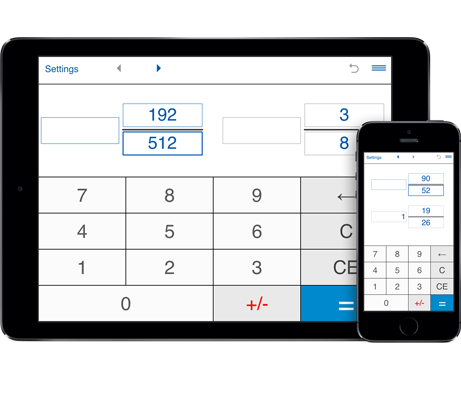# Reduce fractions calculator

A handy calculator that helps you reduce fractions and mixed numbers step by step.
Features:
Reduces fractions to lowest terms.
Supports proper, improper fractions and mixed numbers.
Finds the Greatest Common Factor (GCF) of the numerator and denominator.
Prime factorization of the numerator and denominator.

## Reducing fractions to lowest terms

This reduce fractions calculator will reduce a fraction or mixed number to its lowest terms.
Input the numerator and denominator (interger values).
Click on "=" to reduce it to lowest terms. Fractions are simplified by dividing the numerator and denominator by their greatest common factor.